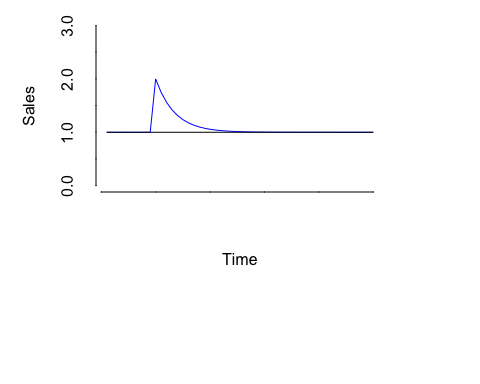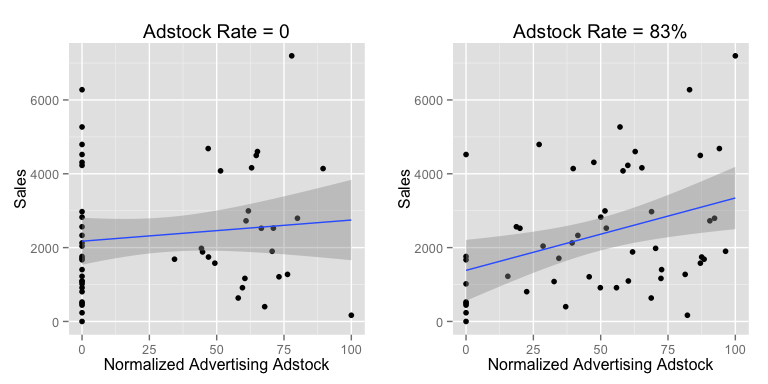## Introduction

Companies have trouble measuring the effectiveness of advertising. This is due primarily to the lag between exposure to an ad and the consumer response to an ad. Simply put, past ads can (and usually do) affect present and future sales. Advertising adstock is a term used to measure the memory effect of advertising carried over from start of advertising. We can then use the advertising adstock as a variable in sales response modeling, e.g. regression analysis.

Visually, the effect of advertising can be thought of as follows:Simon Broadbent developed a frame work for advertising adstock as: $\text{Advertising Adstock}_t = \text{Advertising Adstock}_t + \text{Adstock Rate}\cdot\text{Advertising Adstock}_{t-1}$ where the $$\text{Adstock Rate}$$ is between 0-1 and $$t$$ is a time index.

The adstock can be recursively written in R with the following code:

advertising.adstock = advertising
}

However, a much simpler code can be done with the filter command courtesy of stackoverflow.com:

advertising.adstock = filter(x=advertising, filter=adstock.rate, method="recursive")

Note about normalizing: I normalize data so the range is always between 0-100.

## Visual Exploration of Adstock Effect

Below is an example of two models. One with adstock transfromation and one without. Notice how the regression line fits the data better than the one without adstock.## Building the Model

The power of adstock transfromation is evident during model building. Usually, the model is a regression equation of the form: $\text{Sales}=\alpha + \beta \cdot \text{Adstock}(\text{Advertising}) + \epsilon$

A regression equation's R-Squared can be improved by selecting the appropriate adstock rate. In the previous example, R-Squared for the model with no adstock was 0.0131. The R-Squared for a model with the appropriate adstock rate of 83% was 0.1334. It is not a considerable improvement but brings the point on how to improve the model fit.

Data for this can be found in the Shiny App: http://analyticsartist.shinyapps.io/Adstock_Transformation/.